Courses

# Kinematics (Part - 1) - Mechanics, Irodov JEE Notes | EduRev

## NEET : Kinematics (Part - 1) - Mechanics, Irodov JEE Notes | EduRev

The document Kinematics (Part - 1) - Mechanics, Irodov JEE Notes | EduRev is a part of the NEET Course I. E. Irodov Solutions for Physics Class 11 & Class 12.
All you need of NEET at this link: NEET

Q.1. A motorboat going downstream overcame a raft at a point A; τ = 60 min later it turned back and after some time passed the raft at a distance l = 6.0 km from the point A. Find the flow velocity assuming the duty of the engine to be constant.
Ans. Let, Vbbe the velocity of motorboat
and, vw be the velocity of flow of water.
Let x be the distance travelled down stream by the boat.
Then, x = (vb + vw)τ ................................ (i)
After turning back, suppose the boat takes time t to meet the raft again.
Then, x - I = (vb - vw) t .......................... (ii)
Again, I = vw x (τ + t) ......................... (iii)
Here, vw is the velocity of the raft and (τ + t) is the total time taken by the raft to cover distance I.
From (i), (ii) and (iii) we get:
(vb + Vw)τ - vw (τ + t) = (v6 - vw)t
Solving, τ = f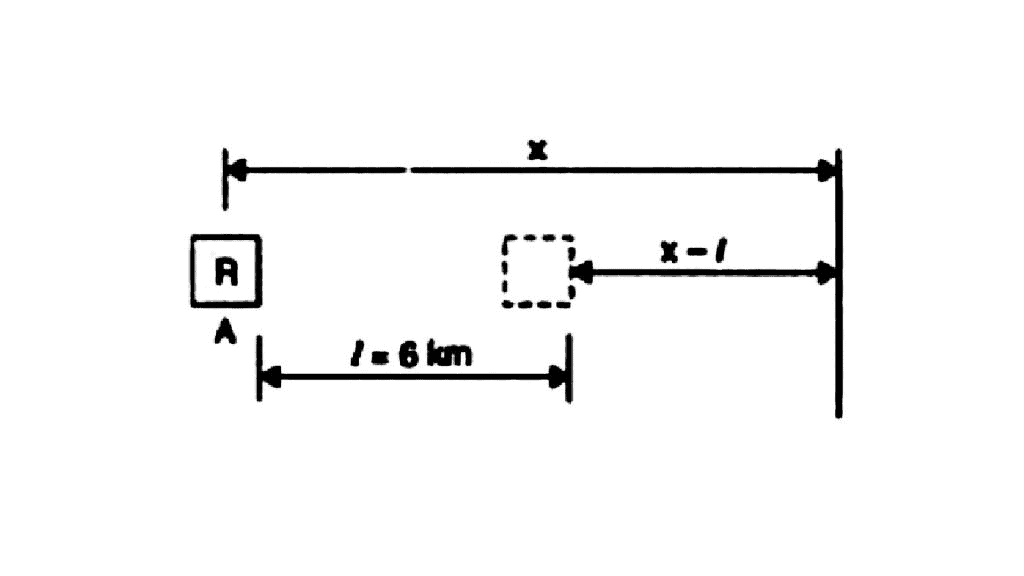From (iii),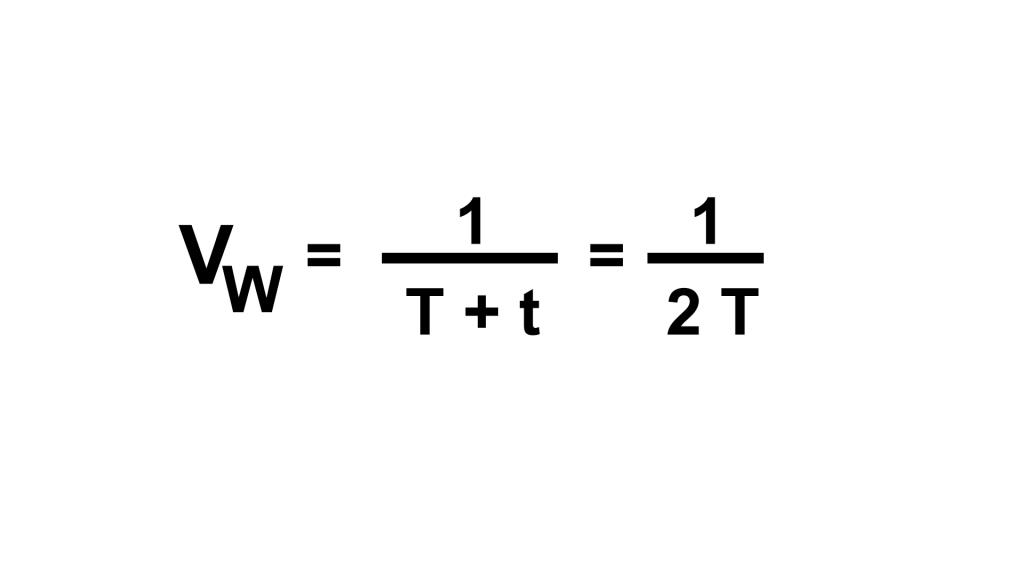Plugging in the values we get,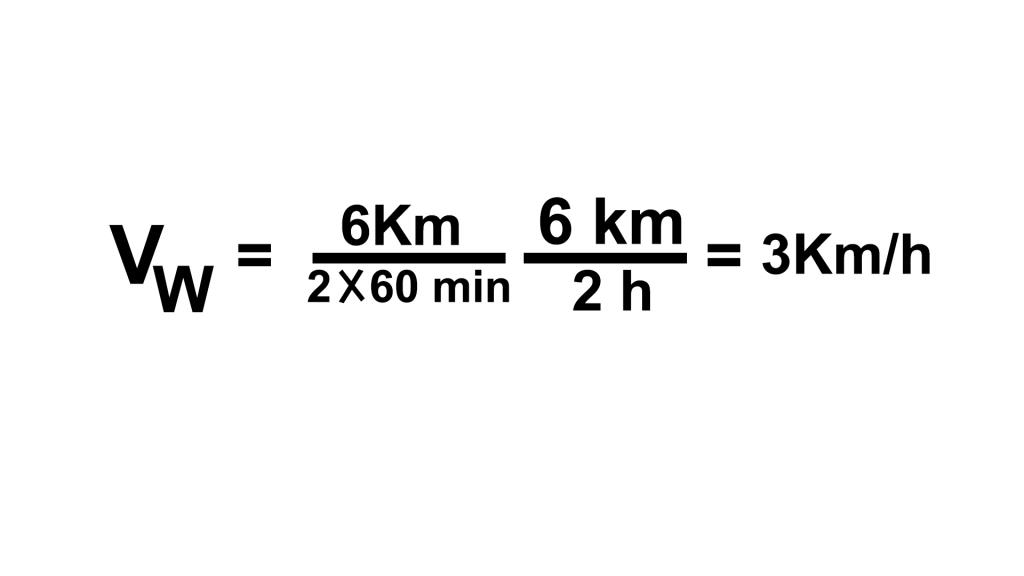Q.2. A point traversed half the distance with a velocity v0. The remaining part of the distance was covered with velocity v1 for half the time, and with velocity v2  for the other half of the time. Find the mean velocity of the point averaged over the whole time of motion.
Ans. Let s be the total distance traversed by the point and t1 the time taken to cover half the distance. Further let 2t be the time to cover the rest half of the distance.

Therefore,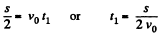(1)

and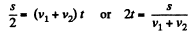(2)

Hence the sought average velocity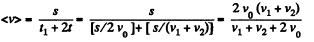Q.3. A car starts moving rectilinearly, first with acceleration ω = 5.0 m/s2  (the initial velocity is equal to zero), then uniformly, and finally, decelerating at the same rate ω, comes to a stop. The total time of motion equals τ = 25 s. The average velocity during that time is equal to (v) = 72 km per hour. How long does the car move uniformly?
Ans.
As the car starts from rest and finally comes to a stop, and the rate of acceleration and deceleration are equal, the distances as well as the times taken are same in these phases of motion.
Let Δt be the time for which the car moves uniformly.
Then the acceleration/deceleration time is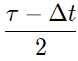each.
So total distance is calculated as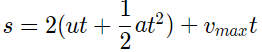vmax  is calculated using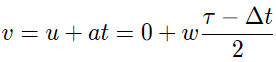Thus we get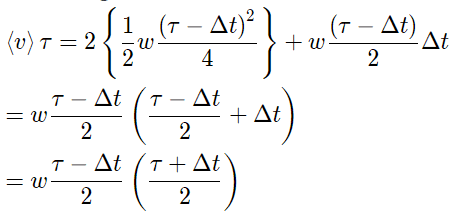Solving it we getHence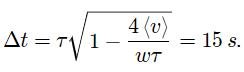Q.4. A point moves rectilinearly in one direction. Fig. 1.1 shows the distance s traversed by the point as a function of the time t. Using the plot find:
(a) the average velocity of the point during the time of motion;
(b) the maximum velocity;
(c) the time moment t0  at which the instantaneous velocity is equal to the mean velocity averaged over the first t0 seconds.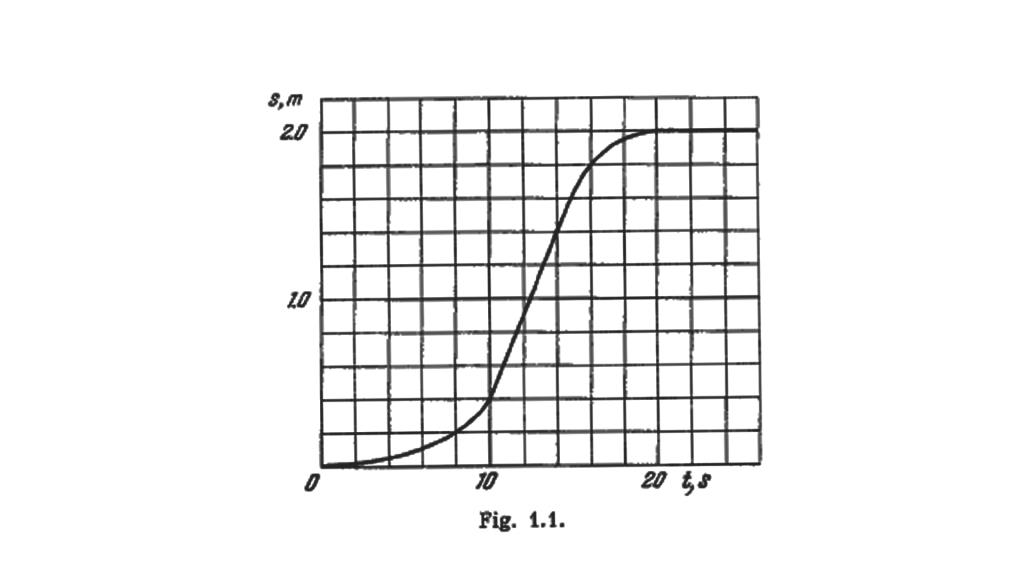Ans.
(a) Sought average velocity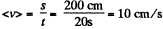(b) For the maximum velocity, ds/dt should be maximum. From the figure ds/dt is maximum for all points on the line ac, thus the sought maximum velocity becomes average velocity for the line ac and is equal to :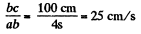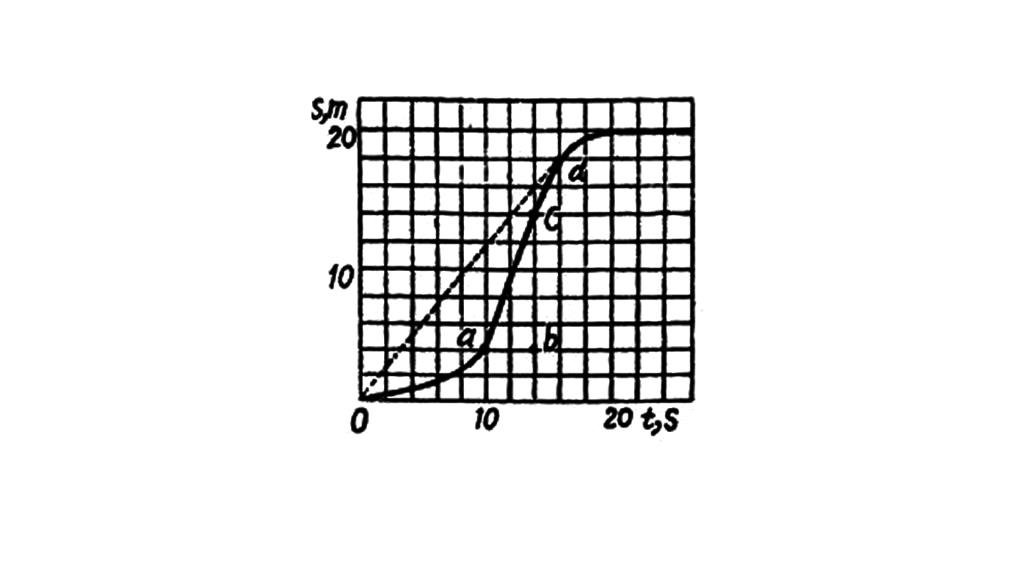(c) Time t0 should be such that corresponding to it the slope ds/dt should pass through the ds s point O (origin), to satisfy the relationship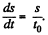From figure the tangent at point d passes through the origin and thus corresponding time t = t0 = 16 s.

Q. 5. Two particles, 1 and 2, move with constant velocities v1 and v2. At the initial moment their radius vectors are equal to r1 and r2. How must these four vectors be interrelated for the particles to collide?
Ans. Let the particles collide at the point A (Fig.), whose position vector is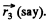If t be the time taken by each particle to reach at point A, from triangle law of vector addition: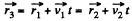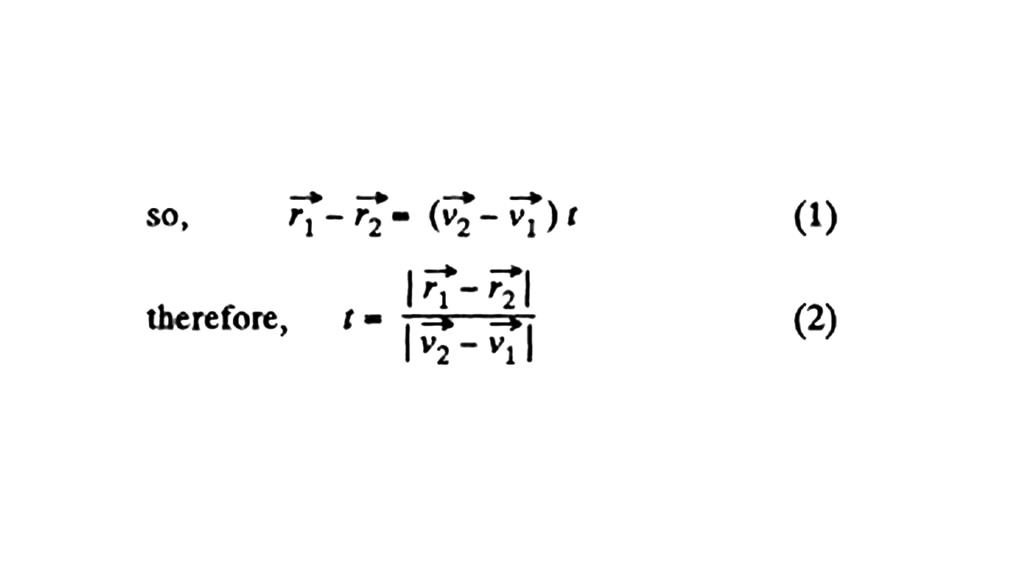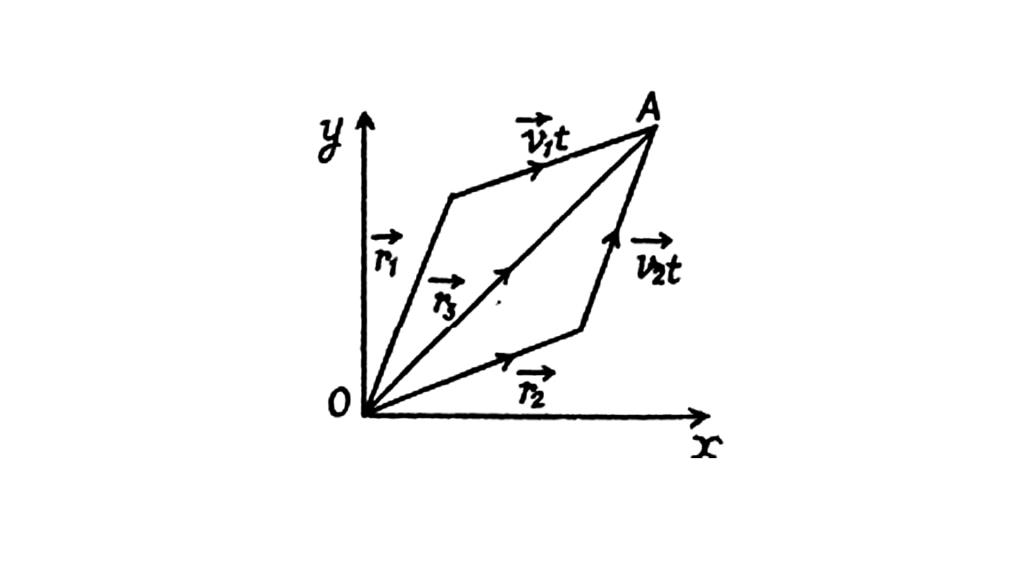From Eqs. (1) and (2)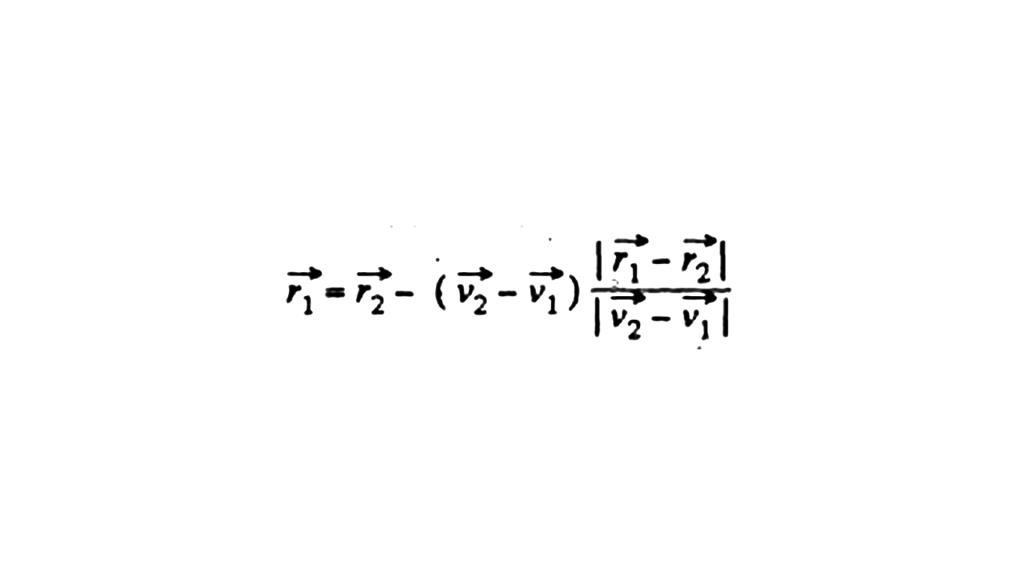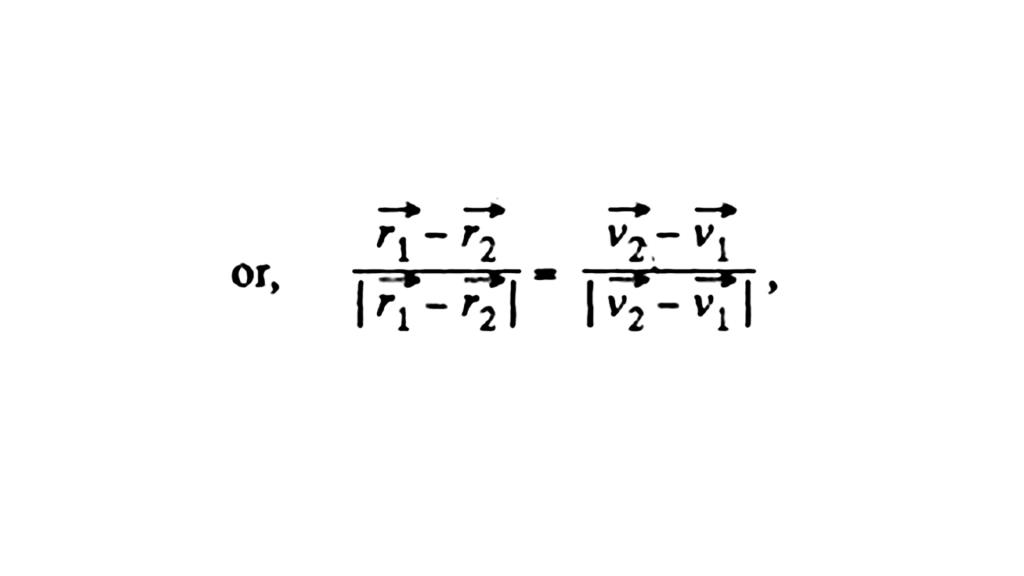which is the sought relationship.

Q.6. A ship moves along the equator to the east with velocity v0  = 30 km/hour. The southeastern wind blows at an angle φ = 60° to the equator with velocity v = 15 km/hour. Find the wind velocity v' relative to the ship and the angle φ' between the equator and the wind direction in the reference frame fixed to the ship.
Ans. We have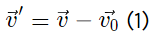From the vector diagram [of equation (1)] and using properties of triangle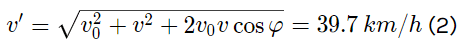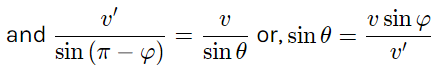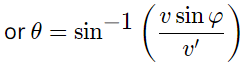Using (2) and putting the values of v and d
θ = 19.1o

Q.7. Two swimmers leave point A on one bank of the river to reach point B lying right across on the other bank. One of them crosses the river along the straight line AB while the other swims at right angles to the stream and then walks the distance that he has been carried away by the stream to get to point B. What was the velocity u of his walking if both swimmers reached the destination simultaneously? The stream velocity v0 = 2.0 km/hour and the velocity if of each swimmer with respect to water equals 2.5 km per hour.
Ans.  Let one of the swimmer (say 1) cross the river along AB, which is obviously the shortest path. Time taken to cross the river by the swimmer 1.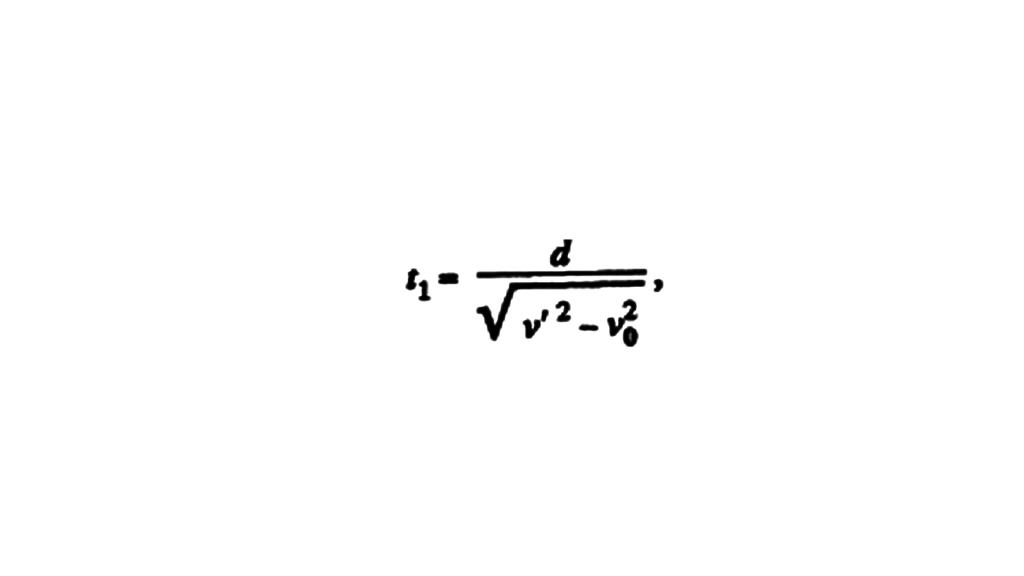(where AB = d is the width of the river).... (1)

For the other swimmer (say 2), which follows the quickest-path, the time taken to cross the river.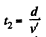.... (2)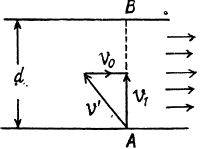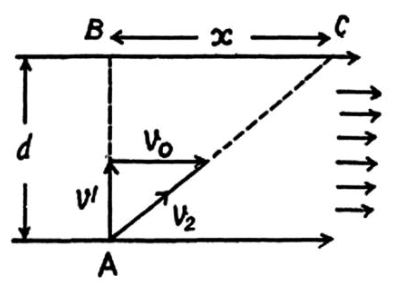In the time t2, drifting of the swimmer 2, becomes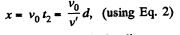(3)

If t3 be the time for swimmer 2 to walk the distance x to come from C to B (Fig.), the(4)

According to the problem t1 = t2 + 13

or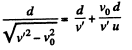On solving we get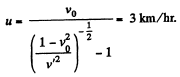Q.8. Two boats, A and B, move away from a buoy anchored at the middle of a river along the mutually perpendicular straight lines: the boat A along the river, and the boat B across the river. Having moved off an equal distance from the buoy the boats returned. Find the ratio of times of motion of boats τAB  if the velocity of each boat with respect to water is η1 = 1.2 times greater than the stream velocity.
Ans.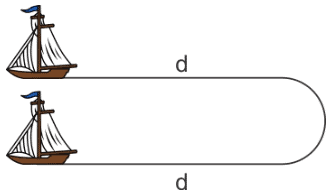For boat A:
Time to reach the same point back is
tA = Time upstream +time downstream =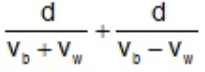Here. vb is the speed of boat with respect to the river vw is the speed of water with respect to Earth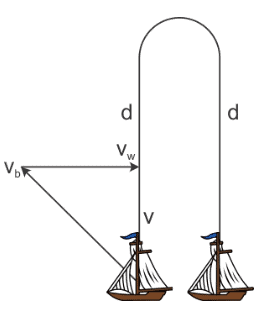For boat B:
The velocity of boat is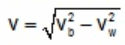Time to reach the same point back is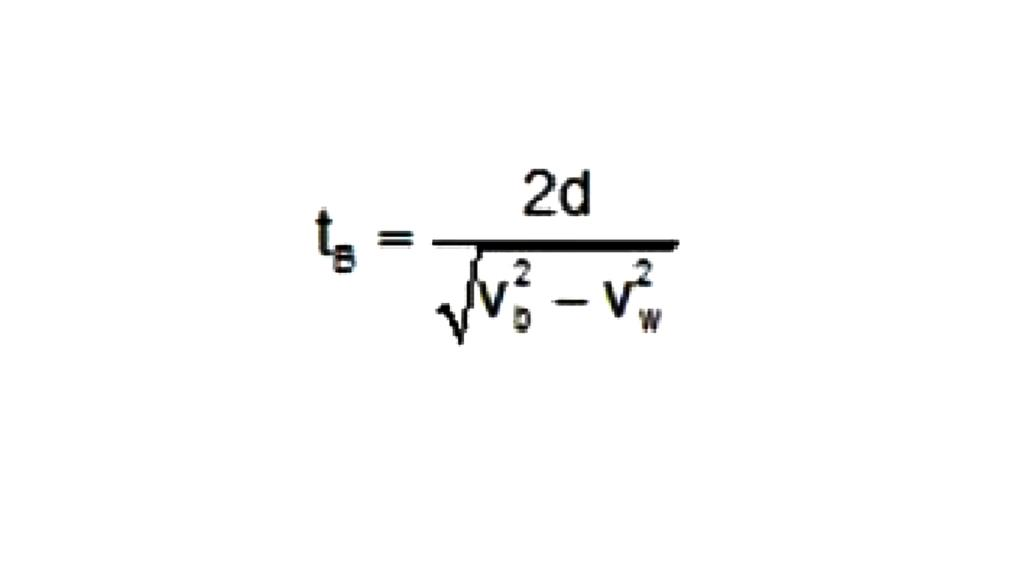Here, vb is the speed of boat with respect to the river vw is the speed of water with respect to Earth
Hence we have the ratio as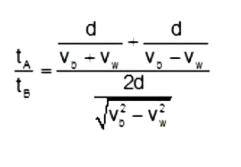It is also given that vb = 1.2vw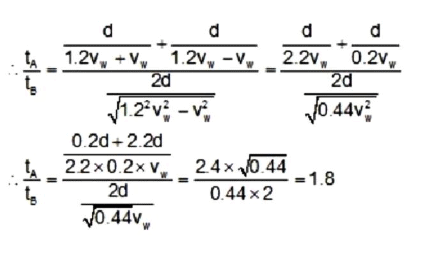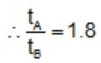Q.9. A boat moves relative to water with a velocity which is n = 2.0 times less than the river flow velocity. At what angle to the stream direction must the boat move to minimize drifting?
Ans. Let v0 be the stream velocity and v' the velocity of boat with respect to water.
At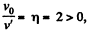some drifting of boat is inevitable.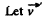make an angle θ with flow direction. (Fig.), then the time taken to cross the river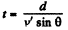(where d is the width of the river)
In this time interval, the drifting of the boat
x = (v' cos θ + v0) t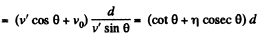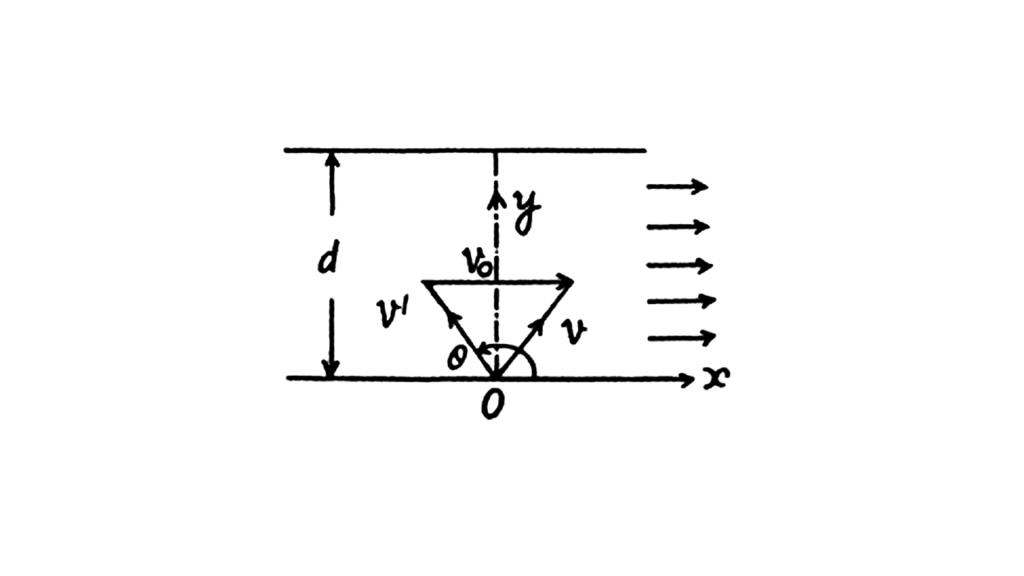For xmin (minimum drifting)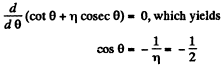Hence, θ = 120°

Q.10. Two bodies were thrown simultaneously from the same point: one, straight up, and the other, at an angle of θ = 60° to the horizontal. The initial velocity of each body is equal to v0  = 25 m/s. Neglecting the air drag, find the distance between the bodies t = 1.70 s later.
Ans. The solution of this problem becomes simple in the frame attached with one of the bodies.
Let the body thrown straight up be 1 and the other body be 2, then for the body 1 in the frame of 2 from the kinematic equation for constant acceleration :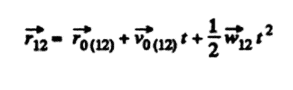So,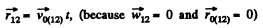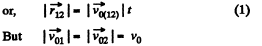So, from properties of triangle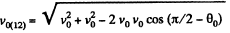Hence, the sought distance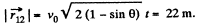Q.11. Two particles move in a uniform gravitational field with an acceleration g. At the initial moment the particles were located at one point and moved with velocities v1 =  3.0 m/s and v2  = 4.0 m/s horizontally in opposite directions. Find the distance between the particles at the moment when their velocity vectors become mutually perpendicular.
Ans. Let the velocities of the paritcles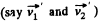becomes mutually perpendicular after time t. Then their velocities become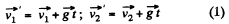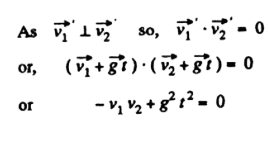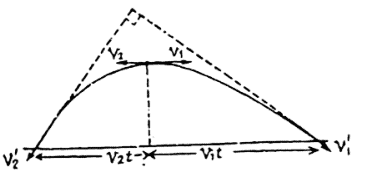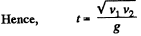.... (3)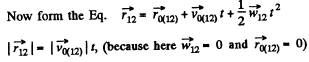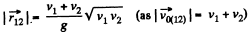Q.12. Three points are located at the vertices of an equilateral triangle whose side equals a. They all start moving simultaneously with velocity v constant in modulus, with the first point heading continually for the second, the second for the third, and the third for the first. How soon will the points converge?
Ans.  From the symmetry of the problem all the three points are always located at the vertices of equilateral triangles of varying side length and finally meet at the centriod of the initial equilateral triangle whose side length is a, in the sought time interval (say t ).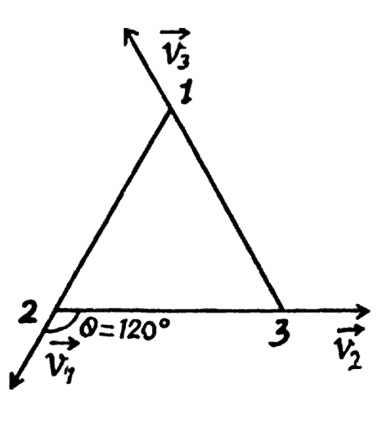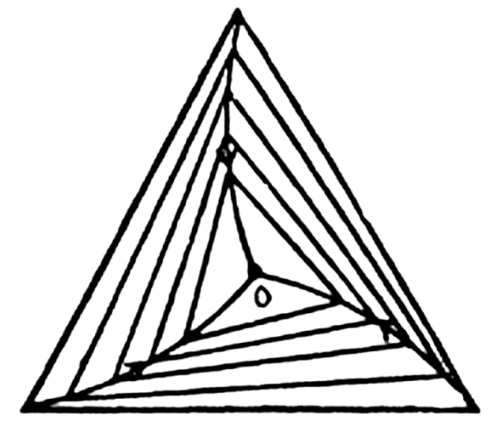Let us consider an arbitrary equilateral triangle of edge length l (say).
Then the rate by which 1 approaches 2, 2 approches 3, and 3 approches 1, becomes: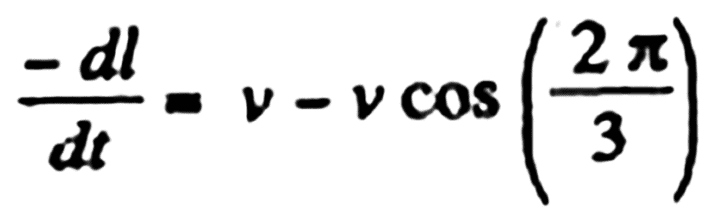On integrating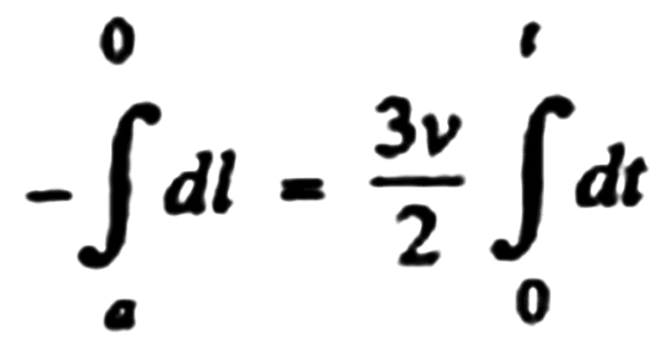Q.13. Point A moves uniformly with velocity v so that the vector v is continually "aimed" at point B which in its turn moves rectilinearly and uniformly with velocity u< v. At the initial moment of time v ⊥ u  and the points are separated by a distance 1. How soon will the points converge?
Ans. Let us locate the points A and B at an arbitrary instant of time (Fig.).
If A and B are separated by the distance s at this moment, then the points converge or point A approaches B with velocity -ds/dt = v - ucosα where angle α  varies with time.

On intergating,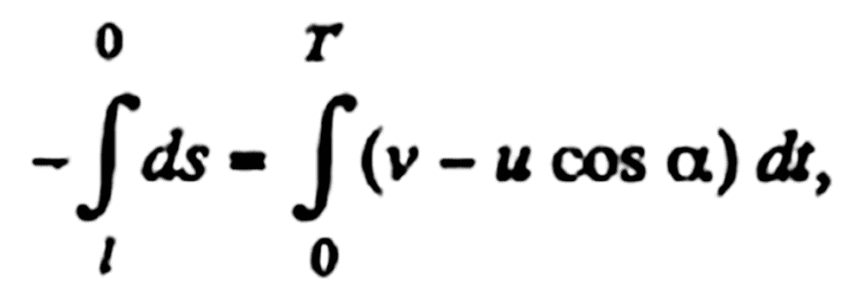(where T is the sought time.)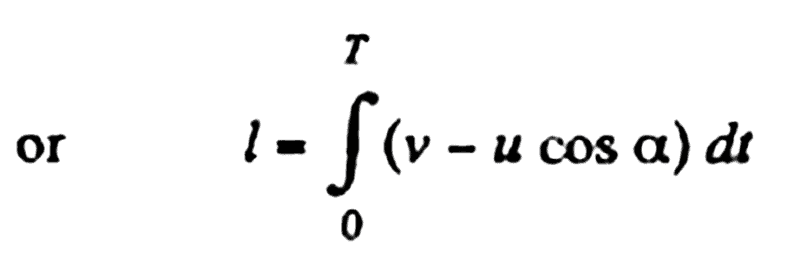(1)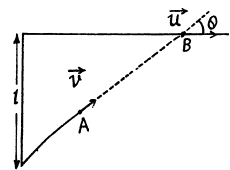As both A and B cover the same distance in jc-direction during the sought time interval, so the other condition which is required, can be obtained by the equation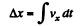So,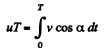(2)

Solving (1) and (2), w e get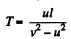One can see that if w = v, or u < v, point A cannot catch B.

Q.14. A train of length l = 350 m starts moving rectilinearly with constant acceleration w = 3.0.10-2  m/s2; t = 30 s after the start the locomotive headlight is switched on (event 1) , and τ = 60 s after that event the tail signal light is switched on (event 2) . Find the distance between these events in the reference frames fixed to the train and to the Earth. How and at what constant velocity V relative to the Earth must a certain reference frame K move for the two events to occur in it at the same point?
Ans. In the reference frame fixed to the train, the distance between the two events is obviously equal to l. Suppose the train starts moving at time t = 0 in the positive x direction and take the origin (x = 0) at the head-light o f the train at t = 0. Then the coordinate o f first event in the earth’s frame is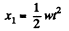and similarly the coordinate of the second event is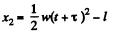The distance between the two events is obviously.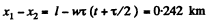in the reference frame fixed on the earth..

For the two events to occur at the same point in the reference frame K, moving with constant velocity V relative to the earth, the distance travelled by the frame in the time interval T must be equal to the above distance.

Thus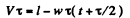So,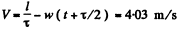The frame K must clearly be moving in a direction opposite to the train so that if (for example) the origin of the frame coincides with the point x1 on the earth at time t, it coincides with the point x2 at time t + τ.

Q. 15. An elevator car whose floor-to-ceiling distance is equal to 2.7 m starts ascending with constant acceleration 1.2 m/s2; 2.0 s after the start a bolt begins falling from the ceiling of the car. Find:

(a) the bolt's free fall time;
(b) the displacement and the distance covered by the bolt during the free fall in the reference frame fixed to the elevator shaft.

Ans. Given that the distance between the elevator floor and ceiling, S = 2.7 m
Acceleration of the elevator, a = 1.2 m/s2.
Relative to the elevator the acceleration of the bolt = (g +a) = ( 9 8+1.2) =11 m/s2
Let 't' be the time taken by the bolt to reach the floor of the elevator of height 2.7 m Relative to the elevator the velocity of the bolt = 0
So from the equation of motion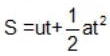Here S = 2.7 m, a = 11 m/s2,u = 0
Substituting we get that: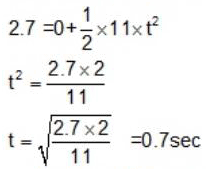∴ The free fall time of the bolt = 0.7 sec
(b)
Displacement:
When the bolt looses its contact from the ceiling of the elevator, it will aquire the velocity of the elevator The upward velocity of the elevator = at = 1.2 x2 = 2 4 m/s
Let us consider the position of elevator shaft as ground.
Therefore in the refrence frame attached to the elevator shaft in the upward direction: The position of the bolt after 0.7s will be: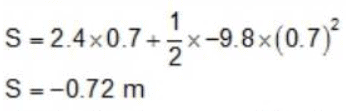So the displacement of the bolt = 0.7 m
Distance:
Distance travelled by the bolt will be different from its displacement as the bolt first travels upwards before it gets detached from the ceiling
The distance travelled upwards can be obtained from v2 = u2 -2gS
From this we get: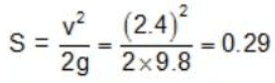The bolt thus has to travel a distance of 2S + 0.7m in total
i.e. 2x0.29 +0.7 =1.3 m

Offer running on EduRev: Apply code STAYHOME200 to get INR 200 off on our premium plan EduRev Infinity!

111 docs

,

,

,

,

,

,

,

,

,

,

,

,

,

,

,

,

,

,

,

,

,

,

,

,

;